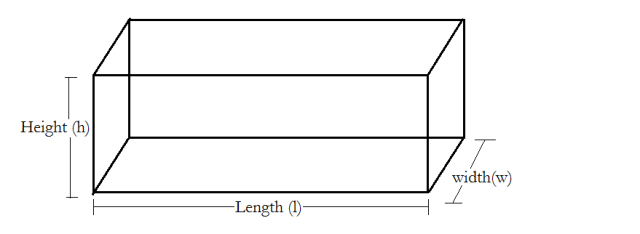# a)Work out the total surface area of this cuboid (3cm x 4cm x 2cm). b) The cuboid has density 7g/cm^3, what is the mass of the cuboid?a) As shown in the diagram above, unlike a cube which has all equal sides, a cuboid can have varying lengths for the height, width and length.

In order to find the total surface area of a cuboid,you need to assess the shape. If you notice, there are 6 faces in total, so basically two of each. So if you find the area of one of the horizontal faces, you can just multiply that by 2 for the total area of the horizontal sides. Same applies for the others. You can even name each face as Face 1, Face 2, and Face 3.

In this case, length is being identified as 3 cm, height is being identified as 4 cm and width as 2 cm. Note that this isn't fixed. It can vary depending on the diagram in the question and other details. Since in this case, they just gave us three different lengths, so you can name either of them as the height, width or length.

Area of Face 1= length×width
=3×2
=6 cm²

Area of Face 2= height×length
=4×3
=12 cm²

Area of Face 3= height×width
=4×2
= 8 cm²

Formula for the Total Surface Area= 2(Area of Face 1) + 2(Area of Face 2) + 2(Area of Face 3)
= 2(6) + 2(12) + 2(8)
=12 + 24 + 16
=52 cm²

b) Density of a Cuboid= Mass÷Volume
Currently, the question has only provided the value of the density. So in order to calculate the mass, we need to calculate the volume first.

Volume of a cuboid= length×height×width
=3×4×2
=24 cm³
Now we substitute this value in the density formula to calculate the mass of this cuboid.

7= Mass÷24
7×24= Mass
Mass= 168 g